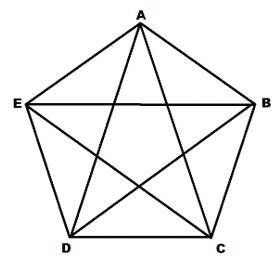Maths-
General
Easy

Question

# Given five noncollinear points, make a conjecture about the number of ways to connect different pairs of points.

## The correct answer is: 4 + 3 + 2 + 1 = 10

### We have given the 5 non-collinear pointsWe have to find the number of ways to connect the different pairs of pointsWe will consider figureWe will consider the points from A to EHere we will consider point APoint A have 4 options to pair, that are B, C, D, E.If we consider point B then,Point B have 3 points to pair that are C, D , E. Because we have considered the pair with A before.If we consider point C then,Point C have 2 points to pair, that are D, E.If we consider point D thenPoint D have 1 point to pair, that are E.Therefore total number of ways the points can be paired is = 4 + 3 + 2 + 1 = 10#### With Turito Foundation.#### Get an Expert Advice From Turito.